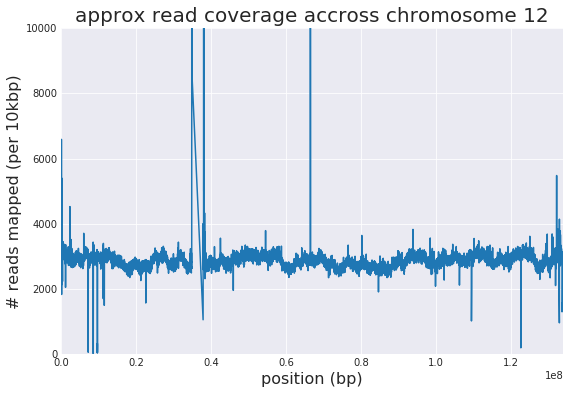# htsget pyspark demo¶

We demonstrate using htsget to load read alignment data into an Apache Spark cluster efficiently, and then further work with it using Spark SQL, all with just a few screenfuls of code.

Warming up:

In [ ]:
%%bash
# install pysam
sudo bash -c "apt-get install -y liblzma-dev libbz2-dev python3-setuptools && easy_install3 pip && pip3 install pysam"
# fetch htsnexus client script
curl -Ls --fail https://raw.githubusercontent.com/dnanexus-rnd/htsnexus/master/client/htsnexus.py > /tmp/htsnexus.py && chmod +x /tmp/htsnexus.py
# fetch regions BED
curl -Ls --fail https://dl.dnanex.us/F/D/6XpKG2jF8g5fQB4JQPxFbZ4K237fx9KzgYYpfF9Z/hs37d5_interLCR_intervals.sorted.4Mbp.bed > /tmp/hs37d5_interLCR_intervals.sorted.4Mbp.bed

In :
# initialize PySpark
import sys, os, subprocess, tempfile, pysam, pyspark
spark = pyspark.SparkContext()

# Utility: Spark accumulator which takes an arbitrary one of the values added to it (or None).
class TakerAccumulatorParam(pyspark.AccumulatorParam):
def zero(self, initialValue):
return None
if v1 is None:
return v2
return v1


Now the main ETL functionality. Use htsget to load NA12878 alignments into a Spark RDD of python dicts. Parallelizing over a given list of genomic regions, call the htsget client to get a BAM slice for each region, then read it using pysam. Owing to the parallelism enabled, this should be much faster than reading the original BAM file from beginning to end.

In :
# In: list of genomic regions as triplets e.g. ("12",111766922,111817529)
# Out: (SAM header, RDD of python dict for each alignment)

alignment_fields = ['cigarstring', 'flag', 'is_duplicate', 'is_paired', 'is_proper_pair', 'is_qcfail',
'mapping_quality', 'mate_is_reverse', 'mate_is_unmapped',
'next_reference_id', 'next_reference_name', 'next_reference_start',
'query_alignment_end', 'query_alignment_length', 'query_alignment_start',
'query_length', 'query_name', 'qual', 'query_sequence',
'reference_end', 'reference_id', 'reference_length', 'reference_name', 'reference_start',
'tags', 'template_length']

def htsget_rdd(regions):

def htsget(region):
fn = tempfile.mkstemp(".bam")
os.close(fn)
cmd = "PYTHONPATH= python2.7 /tmp/htsnexus.py -r {}:{}-{} {} > {}".format(region, region, region, readgroupset, fn)
subprocess.check_call(cmd, shell=True)
return (region, fn)

region, filename = region_and_filename
with pysam.AlignmentFile(filename, "rb") as af:
for aln in af:
# htsget is allowed to return alignments outside of the requested region,
# so filter them here.
if aln.reference_name and aln.reference_name == region \
and aln.reference_start and aln.reference_start <= region \
and aln.reference_end and aln.reference_end >= region:
d = {}
for attr in alignment_fields:
d[attr] = aln.__getattribute__(attr)
yield d

rdd = spark.parallelize(regions).map(htsget).persist().flatMap(lambda fn: parse_alignments(fn, header_taker))
rdd.take(1) # ensure header_taker is populated at least once, given Spark's laziness


Load the RDD of read alignments for chromosomes 12, 17, and 21, using a BED file of convenient <4Mbp regions.

In :
# Load a convenient list of genomic regions (<4Mbp chunks)
regions = []
with open("/tmp/hs37d5_interLCR_intervals.sorted.4Mbp.bed", "r") as bed:
for line in bed:
fields = line.split('\t')
regions.append((fields, int(fields)+1, int(fields)))
# TODO: double-check the +1 vis a vis the overlap filter in parse_alignments
regions = [region for region in regions if region in ['12','17','21']]
len(regions)

Out:
96
In :
%%time
print(alignments.count())

74441320
CPU times: user 128 ms, sys: 6.33 ms, total: 134 ms
Wall time: 1min 52s


Querying the RDD of python dicts: proportion of alignments flagged as secondary.

In :
%time alignments.filter(lambda aln: aln['is_secondary']).count() / alignments.count()

CPU times: user 191 ms, sys: 18.9 ms, total: 209 ms
Wall time: 1min 56s

Out:
0.006543328355810993

We can use the RDD API to parallelize Python-coded algorithms on the alignments.

## SQL Layer¶

Just a few lines of code instantiate a SQL schema & view of the RDD.

In :
import pyspark.sql
spark_sql = pyspark.sql.SparkSession.builder.getOrCreate()
df = spark_sql.createDataFrame(alignments.map(lambda aln: pyspark.sql.Row(**aln)))
df.createOrReplaceTempView("alignments")
df.printSchema()

root
|-- cigarstring: string (nullable = true)
|-- flag: long (nullable = true)
|-- is_duplicate: boolean (nullable = true)
|-- is_paired: boolean (nullable = true)
|-- is_proper_pair: boolean (nullable = true)
|-- is_qcfail: boolean (nullable = true)
|-- is_read1: boolean (nullable = true)
|-- is_read2: boolean (nullable = true)
|-- is_reverse: boolean (nullable = true)
|-- is_secondary: boolean (nullable = true)
|-- is_supplementary: boolean (nullable = true)
|-- is_unmapped: boolean (nullable = true)
|-- mapping_quality: long (nullable = true)
|-- mate_is_reverse: boolean (nullable = true)
|-- mate_is_unmapped: boolean (nullable = true)
|-- next_reference_id: long (nullable = true)
|-- next_reference_name: string (nullable = true)
|-- next_reference_start: long (nullable = true)
|-- qual: string (nullable = true)
|-- query_alignment_end: long (nullable = true)
|-- query_alignment_length: long (nullable = true)
|-- query_alignment_start: long (nullable = true)
|-- query_length: long (nullable = true)
|-- query_name: string (nullable = true)
|-- query_sequence: string (nullable = true)
|-- reference_end: long (nullable = true)
|-- reference_id: long (nullable = true)
|-- reference_length: long (nullable = true)
|-- reference_name: string (nullable = true)
|-- reference_start: long (nullable = true)
|-- tags: array (nullable = true)
|    |-- element: struct (containsNull = true)
|    |    |-- _1: string (nullable = true)
|    |    |-- _2: string (nullable = true)
|-- template_length: long (nullable = true)



Simple SQL query to count primary & secondary alignments on each chromosome.

In :
%%time
spark_sql.sql("\
SELECT reference_name, is_secondary, COUNT(*) FROM alignments WHERE NOT is_duplicate \
GROUP BY reference_id, reference_name, is_secondary ORDER BY reference_id, is_secondary \
").show()

+--------------+------------+--------+
|reference_name|is_secondary|count(1)|
+--------------+------------+--------+
|            12|       false|37549298|
|            12|        true|  142451|
|            17|       false|24219910|
|            17|        true|  127792|
|            21|       false|11138053|
|            21|        true|  216851|
+--------------+------------+--------+

CPU times: user 130 ms, sys: 20.3 ms, total: 150 ms
Wall time: 3min 14s


A more elaborate query, to generate a rough coverage track for chromosome 12 (binning primary alignment start positions by 10kbp)

In :
%%time
rough_coverage = spark_sql.sql("\
SELECT bin, COUNT(*) AS read_count FROM \
(SELECT *, (FLOOR(reference_start/10000)*10000) as bin FROM alignments) AS binned_alignments \
WHERE reference_name = '12' AND is_duplicate = FALSE AND is_secondary = FALSE \
GROUP BY bin ORDER BY bin \
")
rough_coverage = rough_coverage.toPandas()

CPU times: user 214 ms, sys: 5.84 ms, total: 220 ms
Wall time: 3min 18s

In :
import matplotlib.pyplot as plt
import seaborn

seaborn.set_style("darkgrid")
plt.figure(figsize=(9,6))
plt.xlabel("position (bp)", fontsize=16)
plt.ylabel("# reads mapped (per 10kbp)", fontsize=16)
plt.title("approx read coverage accross chromosome 12", fontsize=20)